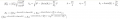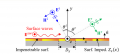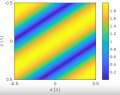# problem creating constractive and destractive interference electromagnetic plot

#### yef smith

Joined Aug 2, 2020
399
Hello , i want to create the plot of E_i and E_r shown bellow , i should get square blocks ,which comes from constructive and distructive interference of the two modes.
I have written the mathematical expressions and implemented them in matlab (code is attached)
but i get in the plot only one wave pattern, no two wave behavior(constructive and distructive interference squares) .Code:
clc
clear all
f=6;
lambda=300/6;
x=linspace(-0.5*lambda,0.5*lambda,1000);
y=linspace(-0.5*lambda,0.5*lambda,1000);
[xx,yy]=meshgrid(x,y);
k=2*pi/lambda;
E_i=1;
eta=377;
D_x=1.064*lambda;
colorbar;